•Calculator Widget Pro

•3.5Current ratings
•\$0.99
•universal
Round up

Age

7 years

Released:2014-11-12

Available in

0 Countries

of 155 total

Activity

0

last updated: n/a

Overall Ratings

6

with average of 3.5

Global Rank

None

Currently not visible

Top 25 Overall

0 Countries

Not in any top 25

Global Rank Positions
Description
Calculator for iPad makes fully use of the new iOS capabilities and puts a normal, scientific and a trigonometric calculator right in your notification center!

FEATURES
* Contains 4 different widgets containing all calculation functions you need. You can choose yourself to add these widgets to your notification center separately. If you, for example, never use sinus and tangs, you can simple remove that widget from your todays view.

WIDGET 1: Calculator
* Quick Equation Preview: Your current Equation is displayed below the Result
* Basic Calculation Buttons
* Percent Button will behave just like the Original Calculator
* Invisible Backspace Button: Slide/Swipe the Result Screen with your finger or use the visible Back Button

* Advanced Mathematical/Scientific buttons like exponent, square, logarithm, etc
* Memory Buttons like the Original Calculator

WIDGET 3: Calculator Trigonometric
* Advanced Trigonometric Mathematical buttons like sinus, cosinus, hyperbolic tangos etc.
* Calculate with Degrees or Radials

WIDGET 4: Calculator History
* History Tape: All calculations are saved and can be copied and forwarded; hide and open with one simple click

CALCULATION FEATURES
The impressive list of mathematical operations you can perform with Calculator Widget Pro includes: Addition, Subtraction, Division, Multiplication, Percentages, Cubic Exponent, Cubic Root, Engineering Exponent, Nth Exponent, Nth Root, Power of E, Power of Ten, Power of Two, Square, Square Root, Natural Logarithm, Common Logarithm, Binary Logarithm, Sinus, Cosinus, Tangus, Hyperbolic Sinus, Hyperbolic Cosinus, Hyperbolic Tangus, Arc Sinus, Arc Cosinus, Arc Tangus, Hyperbolic Arc Sinus, Hyperbolic Arc Cosinus, Hyperbolic Arc Tangus, Multiplicative Inverse, Random Number Input and Factorial.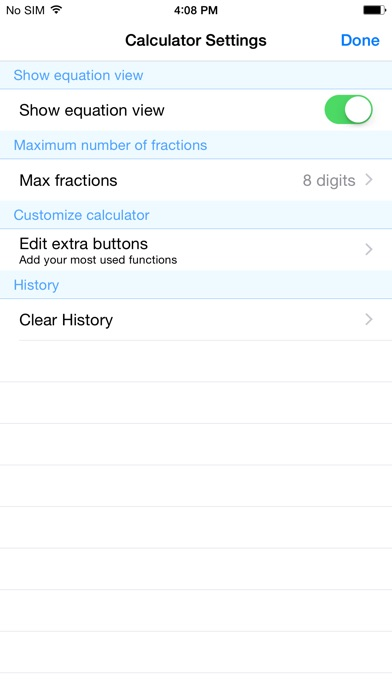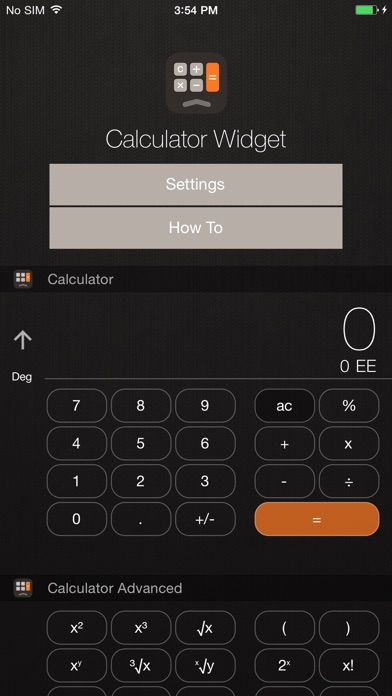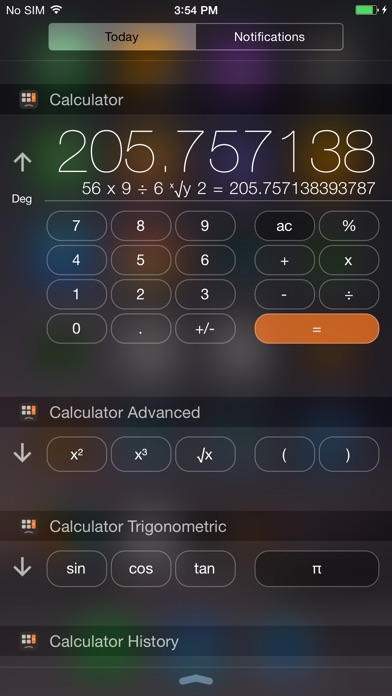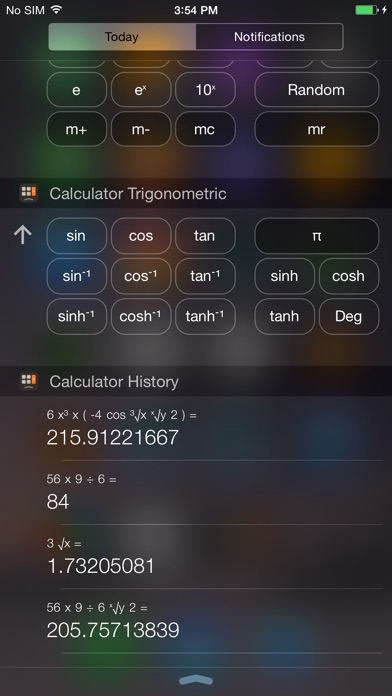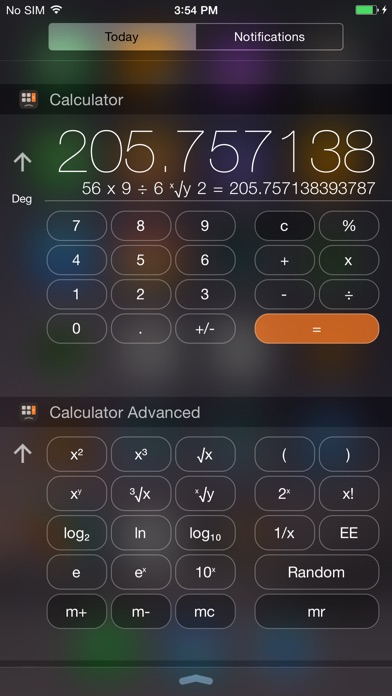3.5 Stars6 ratings